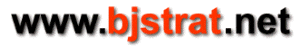## Insurance Count

The Insurance Count is a card counting system that basically measures the density of ten value cards (Ten, Jack, Queen, King) remaining to be dealt. It does so by separating cards into 2 groups, tens and non-tens. All cards are counted. (No cards have a 0 tag.) All ten value cards have the same positive tag and all non-tens have the same negative tag. In blackjack the optional insurance bet when dealer has an ace as the up card pays 2 to 1. If we tag tens as +2 and non-tens as -1 when the running count equals 0 for any shoe composition broken down into tens and non-tens we find that the probability of drawing a ten value card is exactly 1/3. When the probability of drawing a ten value card is 1/3 the expectation for an insurance bet is to win 1 bet for every 2 lost, which means that in the long run this is a break even bet at 2 to 1 odds. When running count exceeds 0 the insurance bet is positive expected value. When running count is less than 0 it is negative expected value.

It would be possible to tag ten value cards and non-tens at different values than +2 and -1 as above as long as each ten value card has the same positive tag and each non-ten has the same negative tag. However, if we do so we will not arrive at the convenient running count value of 0 as the break even point. This is because if the tags are different than +2/-1 the probability of drawing a ten value card when running count is 0 will be something different than 1/3, which is the break even point for an insurance bet. For example if the tags were +1/-1 then when running count is 0 the probability of drawing a ten would be 1/2. (At running count 0 there would be an equal number of tens and non-tens present.) In order to determine the precise probability of drawing a ten value card we would need to know exact number of cards remaining as well as running count in order to compute a running count per deck or true count to make a precise calculation of the probability of drawing a ten value card. Then we could determine the true count that correlates to a 1/3 probability of drawing a ten to define the point at which the insurance bet becomes positive EV. If we choose tags of +2 and -1 as above all we need to know is that if running count exceeds 0, insurance is positive EV, if it equals 0 insurance is an even bet, and if it is less than 0, insurance is negative EV and it is not necessary to compute a true count for this.

The initial running count of the insurance count that tags ten value cards as +2 and non-tens as -1 depends upon the number of decks being used. There are 36 non-tens and 16 tens per deck. Initial running count for a single deck = 36*(-1) + 16*(+2) = -4. Initial running count for any number of decks = (-4)*(number of decks). At the beginning of a shoe the current running count is the initial running count. When a non-ten is dealt current running count is increased by 1. When a ten value card is dealt current running count is decreased by 2. Whenever current running count exceeds 0, insurance is a positive EV bet.

## Shorthand Way to List the Tags of Insurance Count (ranks 1 through 10)

{-1,-1,-1,-1,-1,-1,-1,-1,-1,+2}